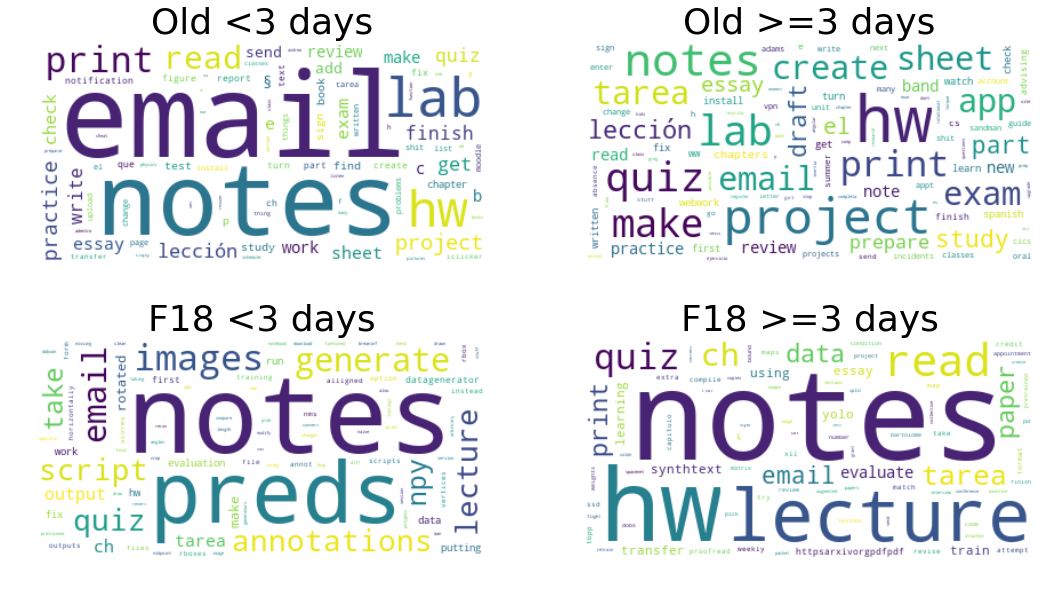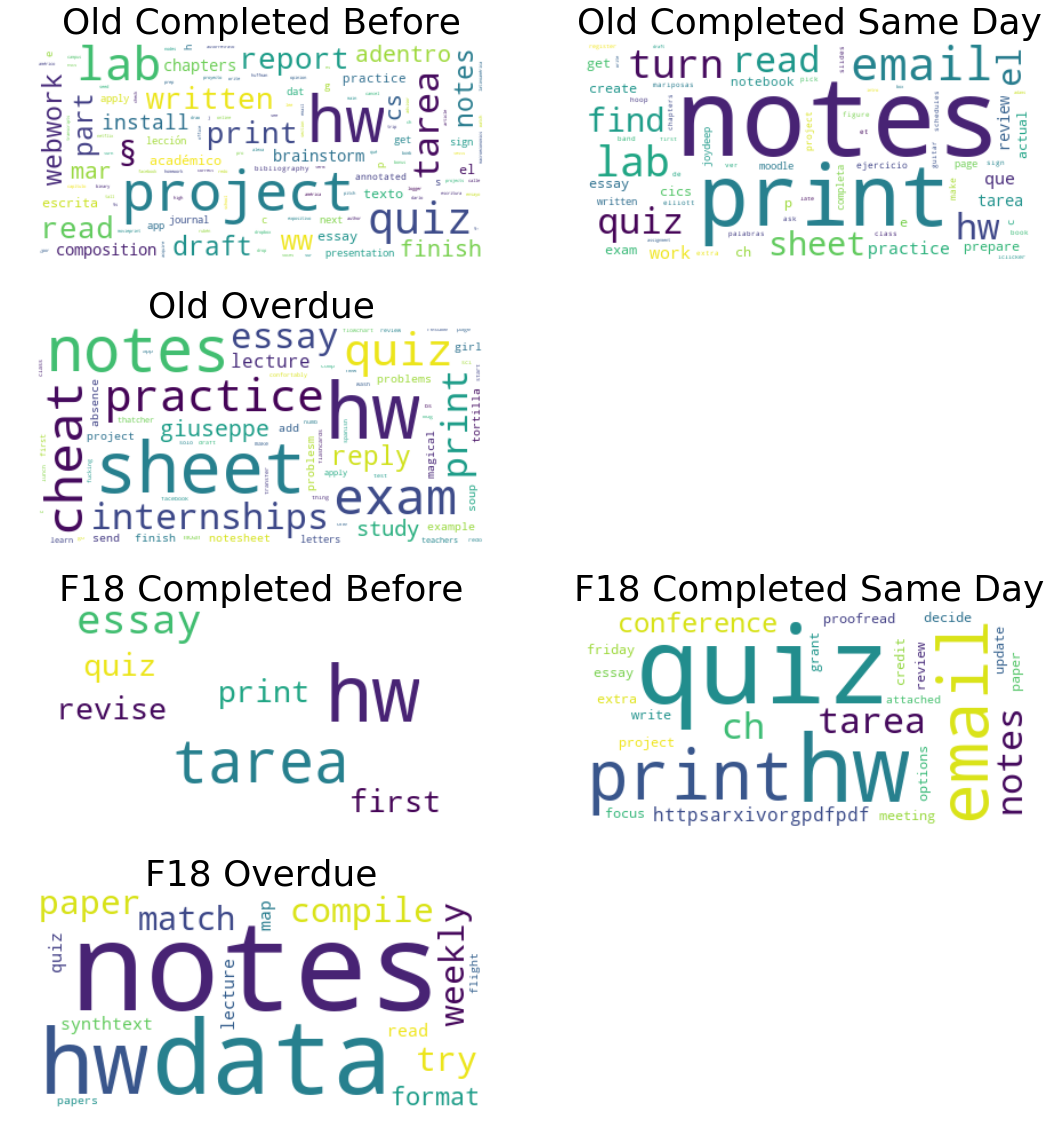Data Analysis

## Asana Data Revisited: Fall 2018 Semester In Review

I previously did a quick analysis of the data from Asana, the website I use to keep track of all my tasks.

Since it’s been about 6 months, I thought it would be interesting to take another look to see which trends remained and which changed.

``````import numpy as np
import pandas as pd
import matplotlib.pyplot as plt
import seaborn as sns
import plotly.graph_objs as go
from plotly import tools
from wordcloud import WordCloud
import datetime
import collections
from lib import custom_utils

init_notebook_mode(connected=True)
``````
``````[nltk_data] Downloading package stopwords to /home/sean/nltk_data...
[nltk_data]   Package stopwords is already up-to-date!
``````

Notice I import a module called `custom_utils`. I recently started a project to keep track of my personal health and development and I found the need to reuse functions often. The file can be found here.

### Data

Unlike last time, I’ll only be pulling the tasks related to school. Just before the start of last semester (Fall 2018), I created a new project using the Boards layout. This allows me to better organize my task by class.

``````f18_df = pd.read_csv('asana-umass-f18.csv', parse_dates=[1, 2, 3, 8, 9])
``````
0 949672124046393 2018-12-17 2018-12-19 2018-12-19 training script to take only outputs for annot... Research NaN NaN NaT NaT NaN NaN UMass NaN
1 949607828976735 2018-12-17 2018-12-18 2018-12-18 make generate preds output .npy file with pred... Research NaN NaN NaT NaT NaN NaN UMass NaN
2 949607828976733 2018-12-17 2018-12-18 2018-12-18 option for generate preds script for not rotat... Research NaN NaN NaT NaT NaN NaN UMass NaN
3 949607828976731 2018-12-17 2018-12-18 2018-12-18 make generate preds scripts output rboxes inst... Research NaN NaN NaT NaT NaN NaN UMass NaN
4 949607828976727 2018-12-17 2018-12-18 2018-12-18 nms Research NaN NaN NaT NaT NaN NaN UMass NaN

Next, we can load the previous data to get some sweet comparison visualizations.

``````old_df = pd.read_csv('School.csv', parse_dates=[1, 2, 3, 7, 8])
old_df.tail()
``````
804 351764138393835 2017-05-29 2017-05-30 2017-05-30 brand new congress questions NaN NaN NaT NaT NaN NaN School NaN
805 351764138393836 2017-05-29 2017-05-29 2017-05-29 sign up for learnupon brand new congress NaN NaN NaT NaT NaN NaN School NaN
806 351764138393838 2017-05-29 2017-06-28 2017-06-28 create base for Hodler app NaN NaN NaT NaT NaN NaN School NaN
807 351779687262554 2017-05-29 2017-06-28 2017-06-28 battery life on lappy ubuntu NaN NaN NaT NaT NaN NaN School NaN
808 356635261007682 2017-06-05 2017-06-28 2017-06-28 bnc volunteer training NaN NaN NaT NaT NaN NaN School NaN
``````all_df = pd.concat([old_df, f18_df], verify_integrity=True, ignore_index=True, sort=True)
``````
0 NaN NaN NaN 2018-04-15 2018-04-15 NaT 2018-04-15 More debt NaN NaN School NaT NaN 148623786710031
1 NaN NaN NaN 2018-04-07 2018-04-06 NaT 2018-04-07 Send one line email to erik to add you to the ... NaN NaN School NaT NaN 148623786710030
2 NaN NaN NaN 2018-03-27 2018-03-27 NaT 2018-03-27 withdraw from study abroad NaN NaN School NaT NaN 610060357624798
3 NaN NaN NaN 2018-03-26 2018-03-09 NaT 2018-03-26 hold NaN NaN School NaT NaN 588106896688257
4 NaN NaN NaN 2018-02-23 2018-02-22 2018-02-23 2018-02-23 find joydeep NaN NaN School NaT NaN 570162249229318

To help retain our sanity, let’s define colors for each semester.

``````all_color = 'rgba(219, 64, 82, 0.7)'
old_color = 'rgba(63, 81, 191, 1.0)'
f18_color = 'rgba(33, 150, 255, 1.0)'
``````

### Task Creation Day of Week Comparison

Let’s see if tasks where still created with the same daily frequencies. Since there are much more tasks in the old data, we can normalize the value counts for a fair comparison. For the sake of keeping the code clean and the x-axis in order, I decided keep the days of the week as numbers. For reference, 0 is Monday.

``````old_df['Created At DOW'] = old_df['Created At'].dt.dayofweek
f18_df['Created At DOW'] = f18_df['Created At'].dt.dayofweek
``````
``````trace1 = go.Bar(
x=old_df['Created At DOW'].value_counts(normalize=True).keys(),
y=old_df['Created At DOW'].value_counts(normalize=True).values,
name='Old Data',
marker={
'color': old_color
}
)
trace2 = go.Bar(
x=f18_df['Created At DOW'].value_counts(normalize=True).keys(),
y=f18_df['Created At DOW'].value_counts(normalize=True).values,
name='Fall 18',
marker={
'color': f18_color
}
)

data = [trace1, trace2]
layout = go.Layout(
barmode='group'
)

fig = go.Figure(data=data, layout=layout)
iplot(fig, filename='DOW Comparison')
``````

This was quite a surprise. The days when I created tasks seems to have changed somewhat this semester.

Let’s check if the overall trend has remained the same.

``````all_df['Created At DOW'] = all_df['Created At'].dt.dayofweek

trace1 = go.Bar(
x=all_df['Created At DOW'].value_counts(normalize=True).keys(),
y=all_df['Created At DOW'].value_counts(normalize=True).values,
name='All Data',
marker={
'color': all_color
}
)

data = [trace1]
layout = go.Layout(
barmode='group'
)

fig = go.Figure(data=data, layout=layout)
iplot(fig, filename='DOW Comparison')
``````

There are definitely some small changes. Thursday caught up to Wednesday and Monday is catching up to Tuesday. However, the overall trend that I create the majority of my tasks at the beginning of the week remains strong.

### Completion Time

Next, let’s look at the duration it took for me to complete each task. Because I used the `parse_dates` parameter when importing the CSVs, using the minus operator will return timedelta objects. Since Asana only provided dates without time, tasks with a duration of 0 days are ones that were created and completed on the same day.

Having already found outliers in the last analysis, let’s only consider only tasks that took less than 30 days to complete. Again, we normalize for a better comparison.

``````old_df['Duration'] = (old_df['Completed At'] - old_df['Created At'])
f18_df['Duration'] = (f18_df['Completed At'] - f18_df['Created At'])
``````
``````trace1 = go.Bar(
x=old_df[(old_df['Duration'].astype('timedelta64[D]') < 30)]['Duration'].value_counts(normalize=True).keys().days,
y=old_df[(old_df['Duration'].astype('timedelta64[D]') < 30)]['Duration'].value_counts(normalize=True).values,
name='Old Data',
marker={
'color': old_color
}
)
trace2 = go.Bar(
x=f18_df[(f18_df['Duration'].astype('timedelta64[D]') < 30)]['Duration'].value_counts(normalize=True).keys().days,
y=f18_df[(f18_df['Duration'].astype('timedelta64[D]') < 30)]['Duration'].value_counts(normalize=True).values,
name='Fall 18',
marker={
'color': f18_color
}
)
data = [trace1, trace2]
layout = go.Layout(
barmode='group'
)

fig = go.Figure(data=data, layout=layout)
iplot(fig, filename='grouped-bar')
``````

Now this is interesting! For the most part, the time it takes me to complete tasks seems to have remained relatively the same. However, this semester it seems I create more tasks that take around a week to complete. In addition, I made less tasks that were completed on the same day.

Next, like last time, let’s see if we can figure out what type of tasks usually take longer to complete. I will once again use the fantastic word_cloud library by amueller.

``````# concatenate all name fields from tasks separated by duration of 3 days
old_less_text = ' '.join(list(old_df[old_df['Duration'].astype('timedelta64[D]') < 3]['Name'].dropna()))
old_grtr_text = ' '.join(list(old_df[old_df['Duration'].astype('timedelta64[D]') >= 3]['Name'].dropna()))

f18_less_text = ' '.join(list(f18_df[f18_df['Duration'].astype('timedelta64[D]') < 3]['Name'].dropna()))
f18_grtr_text = ' '.join(list(f18_df[f18_df['Duration'].astype('timedelta64[D]') >= 3]['Name'].dropna()))

# prep text
old_less_text = custom_utils.prep_text_for_wordcloud(old_less_text)
old_grtr_text = custom_utils.prep_text_for_wordcloud(old_grtr_text)

f18_less_text = custom_utils.prep_text_for_wordcloud(f18_less_text)
f18_grtr_text = custom_utils.prep_text_for_wordcloud(f18_grtr_text)

# get word frequencies
old_less_counts = dict(collections.Counter(old_less_text.split()))
old_grtr_counts = dict(collections.Counter(old_grtr_text.split()))

f18_less_counts = dict(collections.Counter(f18_less_text.split()))
f18_grtr_counts = dict(collections.Counter(f18_grtr_text.split()))

# create wordclouds
old_less_wordcloud = WordCloud(background_color="white", max_words=1000, margin=10,random_state=1).generate_from_frequencies(old_less_counts)
old_grtr_wordcloud = WordCloud(background_color="white", max_words=1000, margin=10,random_state=1).generate_from_frequencies(old_grtr_counts)

f18_less_wordcloud = WordCloud(background_color="white", max_words=1000, margin=10,random_state=1).generate_from_frequencies(f18_less_counts)
f18_grtr_wordcloud = WordCloud(background_color="white", max_words=1000, margin=10,random_state=1).generate_from_frequencies(f18_grtr_counts)

# display wordclouds using matplotlib
f, axes = plt.subplots(2, 2, sharex=True)
f.set_size_inches(18, 10)
axes[0, 0].imshow(old_less_wordcloud, interpolation="bilinear")
axes[0, 0].set_title('Old <3 days', fontsize=36)
axes[0, 0].axis("off")
axes[0, 1].imshow(old_grtr_wordcloud, interpolation="bilinear")
axes[0, 1].set_title('Old >=3 days', fontsize=36)
axes[0, 1].axis("off")

axes[1, 0].imshow(f18_less_wordcloud, interpolation="bilinear")
axes[1, 0].set_title('F18 <3 days', fontsize=36)
axes[1, 0].axis("off")
axes[1, 1].imshow(f18_grtr_wordcloud, interpolation="bilinear")
axes[1, 1].set_title('F18 >=3 days', fontsize=36)
axes[1, 1].axis("off")
``````
``````(-0.5, 399.5, 199.5, -0.5)
``````A few things changed this semester. The research project I was on during the semester was pretty demanding so a lot of those tasks show up like `image`, `preds`, or `synthtext`. Also, since none of my classes had projects this semester, that doesn’t show up.

However, some things remained the same. Homework usually takes more than 3 days and lecture notes are either done quickly or they get put off because other tasks are more important.

Next, let’s take a look at overdue tasks.

``````old_df['Overdue'] = old_df['Completed At'] - old_df['Due Date']
f18_df['Overdue'] = f18_df['Completed At'] - f18_df['Due Date']
``````
``````trace1 = go.Bar(
x=old_df['Overdue'].value_counts(normalize=True).keys().days,
y=old_df['Overdue'].value_counts(normalize=True).values,
name='Old Data',
marker={
'color': old_color
}
)
trace2 = go.Bar(
x=f18_df['Overdue'].value_counts(normalize=True).keys().days,
y=f18_df['Overdue'].value_counts(normalize=True).values,
name='Fall 18',
marker={
'color': f18_color
}
)
data = [trace1, trace2]
layout = go.Layout(
barmode='group'
)

fig = go.Figure(data=data, layout=layout)
iplot(fig, filename='grouped-bar')
``````

Seems like I did alright staying on top of things this semester.

Again, let’s use wordclouds to check out what might be causing me to miss due dates.

``````# concatenate all name fields from overdue tasks
old_before_text = ' '.join(list(old_df[old_df['Overdue'].astype('timedelta64[D]') < 0]['Name'].dropna()))
old_sameday_text = ' '.join(list(old_df[old_df['Overdue'].astype('timedelta64[D]') == 0]['Name'].dropna()))
old_overdue_text = ' '.join(list(old_df[old_df['Overdue'].astype('timedelta64[D]') > 0]['Name'].dropna()))

f18_before_text = ' '.join(list(f18_df[f18_df['Overdue'].astype('timedelta64[D]') < 0]['Name'].dropna()))
f18_sameday_text = ' '.join(list(f18_df[f18_df['Overdue'].astype('timedelta64[D]') == 0]['Name'].dropna()))
f18_overdue_text = ' '.join(list(f18_df[f18_df['Overdue'].astype('timedelta64[D]') > 0]['Name'].dropna()))

# prep text
old_before_text = custom_utils.prep_text_for_wordcloud(old_before_text)
old_sameday_text = custom_utils.prep_text_for_wordcloud(old_sameday_text)
old_overdue_text = custom_utils.prep_text_for_wordcloud(old_overdue_text)

f18_before_text = custom_utils.prep_text_for_wordcloud(f18_before_text)
f18_sameday_text = custom_utils.prep_text_for_wordcloud(f18_sameday_text)
f18_overdue_text = custom_utils.prep_text_for_wordcloud(f18_overdue_text)

# get word frequencies
old_before_counts = dict(collections.Counter(old_before_text.split()))
old_sameday_counts = dict(collections.Counter(old_sameday_text.split()))
old_overdue_counts = dict(collections.Counter(old_overdue_text.split()))

f18_before_counts = dict(collections.Counter(f18_before_text.split()))
f18_sameday_counts = dict(collections.Counter(f18_sameday_text.split()))
f18_overdue_counts = dict(collections.Counter(f18_overdue_text.split()))

# create wordclouds
old_before_wordcloud = WordCloud(background_color="white", max_words=1000, margin=10,random_state=1).generate_from_frequencies(old_before_counts)
old_sameday_wordcloud = WordCloud(background_color="white", max_words=1000, margin=10,random_state=1).generate_from_frequencies(old_sameday_counts)
old_overdue_wordcloud = WordCloud(background_color="white", max_words=1000, margin=10,random_state=1).generate_from_frequencies(old_overdue_counts)

f18_before_wordcloud = WordCloud(background_color="white", max_words=1000, margin=10,random_state=1).generate_from_frequencies(f18_before_counts)
f18_sameday_wordcloud = WordCloud(background_color="white", max_words=1000, margin=10,random_state=1).generate_from_frequencies(f18_sameday_counts)
f18_overdue_wordcloud = WordCloud(background_color="white", max_words=1000, margin=10,random_state=1).generate_from_frequencies(f18_overdue_counts)

# display wordclouds using matplotlib
f, axes = plt.subplots(4, 2, sharex=True)
f.set_size_inches(18, 20)
axes[0, 0].imshow(old_before_wordcloud, interpolation="bilinear")
axes[0, 0].set_title('Old Completed Before', fontsize=36)
axes[0, 0].axis("off")
axes[0, 1].imshow(old_sameday_wordcloud, interpolation="bilinear")
axes[0, 1].set_title('Old Completed Same Day', fontsize=36)
axes[0, 1].axis("off")
axes[1, 0].imshow(old_overdue_wordcloud, interpolation="bilinear")
axes[1, 0].set_title('Old Overdue', fontsize=36)
axes[1, 0].axis("off")
axes[1, 1].axis("off")

axes[2, 0].imshow(f18_before_wordcloud, interpolation="bilinear")
axes[2, 0].set_title('F18 Completed Before', fontsize=36)
axes[2, 0].axis("off")
axes[2, 1].imshow(f18_sameday_wordcloud, interpolation="bilinear")
axes[2, 1].set_title('F18 Completed Same Day', fontsize=36)
axes[2, 1].axis("off")
axes[3, 0].imshow(f18_overdue_wordcloud, interpolation="bilinear")
axes[3, 0].set_title('F18 Overdue', fontsize=36)
axes[3, 0].axis("off")
axes[3, 1].axis("off")
``````
``````(-0.5, 399.5, 0.0, 1.0)
``````## Busiest Class this Semester

Since I began to track the class for each task, we can check out which class was my busiest this semester.

``````# https://community.plot.ly/t/setting-up-pie-charts-subplots-with-an-appropriate-size-and-spacing/5066
domain1={'x': [0, 1], 'y': [0, 1]}#cell (1,1)

fig = {
"data": [
{
"values": f18_df['Column'].value_counts().values,
"labels": f18_df['Column'].value_counts().keys(),
'domain': domain1,
"name": "Fall 18",
"hoverinfo":"label+percent+name",
"hole": .4,
"type": "pie"
}],
"layout": {
"annotations": [
{
"font": {
"size": 15
},
"showarrow": False,
"text": "Fall 2018",
"x": 0.5,
"y": 0.5
}
]
}
}

iplot(fig, filename='donut')
``````

## Due Date Frequency this Semester

We can also see when my tasks were due.

``````trace1 = go.Bar(
x=f18_df['Due Date'].dropna().value_counts().keys(),
y=f18_df['Due Date'].dropna().value_counts().values,
name='Fall 18',
marker={
'color': f18_color
}
)

data = [trace1]
iplot(data, filename='due date freq')
``````

## Conclusion

Hopefully this post show the motivation and potential benefit of revisiting a previous analysis in an attempt to find any significant changes. In the context of personal development, doing so can help you track your progress and achieve your goals. I still definitely need to put more effort into taking notes on time!